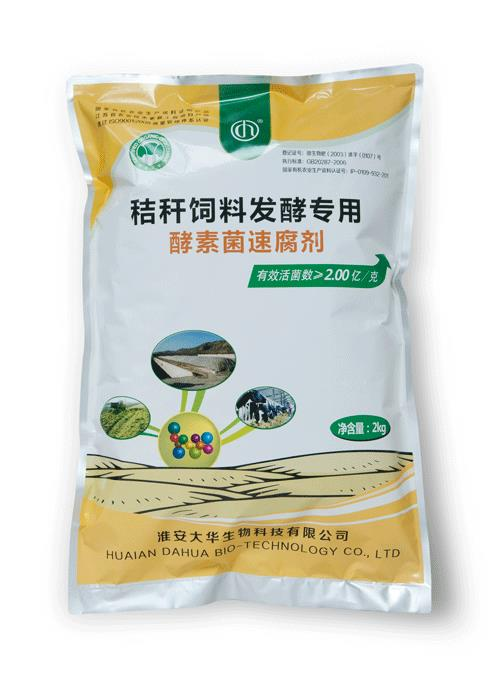• 水产专用
• 大量元素水溶肥料
• 含腐殖酸水溶肥料
• 含氨基酸水溶肥料
• 发酵菌剂
• 沼气发酵菌剂
• 肥水专用
• 净水剂
• 发酵增产剂
• 生物反应堆菌剂
• 秸秆饲料发酵剂
• 酵素菌速腐剂
• 废菌料发酵剂
• 育苗基质
• 水稻育苗基质
• 蓝莓栽培基质
• 蔬菜育苗基质
• 生物有机肥
• 有机肥料
• 生物有机肥
• 食用菌专用
• 发酵增产剂
• 菇宝乐
• 联系我们
• 产品分类
• 发酵菌剂
• 生物反应堆菌剂
• 沼气发酵菌剂
• 肥水专用
• 发酵增产剂
• 秸秆饲料发酵剂
• 废菌料发酵剂
• 酵素菌速腐剂
• 净水剂
• 水溶肥料
• 大量元素水溶肥料
• 含腐殖酸水溶肥料
• 含氨基酸水溶肥料
• 育苗基质
• 蓝莓栽培基质
• 蔬菜育苗基质
• 水稻育苗基质
• 生物有机肥
• 有机肥料
• 生物有机肥
• 水产制剂
• 水产专用
• 食用菌专用
• 菇宝乐
• 发酵增产剂
• 园艺资材
• 有机肥料
• 水稻育苗基质
• 花盆
• 含氨基酸水溶肥料
• 菇宝乐
• 发酵增产剂
• 土壤修复剂
• 土壤调理剂
• 产品分类
• 发酵菌剂
• 水溶肥料
• 育苗基质
• 生物有机肥
• 食用菌专用
• 园艺资材
• 土壤调理剂
• 土壤修复剂
• 水产制剂
• 产品分类
• 土壤调理剂
• 土壤修复剂
• 水产制剂
• 水产专用
• 育苗基质
• 蓝莓栽培基质
• 蔬菜育苗基质
• 水稻育苗基质
• 发酵菌剂
• 发酵增产剂
• 肥水专用
• 沼气发酵菌剂
• 净水剂
• 生物反应堆菌剂
• 秸秆饲料发酵剂
• 废菌料发酵剂
• 酵素菌速腐剂
• 水溶肥料
• 大量元素水溶肥料
• 含氨基酸水溶肥料
• 含腐殖酸水溶肥料
• 生物有机肥
• 有机肥料
• 生物有机肥
• 食用菌专用
• 菇宝乐
• 发酵增产剂
• 园艺资材
• 菇宝乐
• 发酵增产剂
• 花盆
• 水稻育苗基质
• 含氨基酸水溶肥料
• 有机肥料
• 产品分类
• 发酵菌剂
• 发酵增产剂
• 沼气发酵菌剂
• 净水剂
• 生物反应堆菌剂
• 秸秆饲料发酵剂
• 废菌料发酵剂
• 酵素菌速腐剂
• 肥水专用
• 水溶肥料
• 大量元素水溶肥料
• 含腐殖酸水溶肥料
• 含氨基酸水溶肥料
• 育苗基质
• 蓝莓栽培基质
• 蔬菜育苗基质
• 水稻育苗基质
• 生物有机肥
• 有机肥料
• 生物有机肥
• 土壤调理剂
• 食用菌专用
• 菇宝乐
• 发酵增产剂
• 园艺资材
• 有机肥料
• 水稻育苗基质
• 花盆
• 含氨基酸水溶肥料
• 发酵增产剂
• 菇宝乐
• 土壤修复剂
• 水产制剂
• 水产专用
• 产品分类
• 土壤调理剂
• 土壤修复剂
• 水产制剂
• 水产专用
• 发酵菌剂
• 沼气发酵菌剂
• 肥水专用
• 净水剂
• 发酵增产剂
• 生物反应堆菌剂
• 秸秆饲料发酵剂
• 废菌料发酵剂
• 酵素菌速腐剂
• 水溶肥料
• 含氨基酸水溶肥料
• 含腐殖酸水溶肥料
• 大量元素水溶肥料
• 育苗基质
• 蓝莓栽培基质
• 蔬菜育苗基质
• 水稻育苗基质
• 生物有机肥
• 有机肥料
• 生物有机肥
• 食用菌专用
• 菇宝乐
• 发酵增产剂
• 园艺资材
• 含氨基酸水溶肥料
• 菇宝乐
• 发酵增产剂
• 水稻育苗基质
• 有机肥料
• 花盆
• 网站首页
• 关于我们
• 总经理致辞
• 企业文化
• 组织架构
• 公司概况
• 产品分类
• 土壤调理剂
• 水产制剂
• 水产专用
• 园艺资材
• 有机肥料
• 水稻育苗基质
• 花盆
• 含氨基酸水溶肥料
• 菇宝乐
• 发酵增产剂
• 育苗基质
• 蓝莓栽培基质
• 蔬菜育苗基质
• 水稻育苗基质
• 生物有机肥
• 有机肥料
• 生物有机肥
• 食用菌专用
• 发酵增产剂
• 菇宝乐
• 土壤修复剂
• 水溶肥料
• 大量元素水溶肥料
• 含腐殖酸水溶肥料
• 含氨基酸水溶肥料
• 发酵菌剂
• 废菌料发酵剂
• 酵素菌速腐剂
• 沼气发酵菌剂
• 肥水专用
• 净水剂
• 发酵增产剂
• 生物反应堆菌剂
• 秸秆饲料发酵剂
• 产品分类
• 发酵菌剂
• 沼气发酵菌剂
• 肥水专用
• 净水剂
• 发酵增产剂
• 生物反应堆菌剂
• 秸秆饲料发酵剂
• 废菌料发酵剂
• 酵素菌速腐剂
• 水溶肥料
• 大量元素水溶肥料
• 含腐殖酸水溶肥料
• 含氨基酸水溶肥料
• 育苗基质
• 蓝莓栽培基质
• 蔬菜育苗基质
• 水稻育苗基质
• 生物有机肥
• 有机肥料
• 生物有机肥
• 食用菌专用
• 菇宝乐
• 发酵增产剂
• 园艺资材
• 含氨基酸水溶肥料
• 有机肥料
• 花盆
• 菇宝乐
• 发酵增产剂
• 水稻育苗基质
• 土壤调理剂
• 土壤修复剂
• 水产制剂
• 水产专用
• 产品分类
• 园艺资材
• 有机肥料
• 水稻育苗基质
• 花盆
• 含氨基酸水溶肥料
• 菇宝乐
• 发酵增产剂
• 土壤调理剂
• 水产制剂
• 水产专用
• 土壤修复剂
• 发酵菌剂
• 生物反应堆菌剂
• 沼气发酵菌剂
• 肥水专用
• 发酵增产剂
• 秸秆饲料发酵剂
• 废菌料发酵剂
• 酵素菌速腐剂
• 净水剂
• 食用菌专用
• 菇宝乐
• 发酵增产剂
• 水溶肥料
• 大量元素水溶肥料
• 含腐殖酸水溶肥料
• 含氨基酸水溶肥料
• 育苗基质
• 蓝莓栽培基质
• 蔬菜育苗基质
• 水稻育苗基质
• 生物有机肥
• 生物有机肥
• 有机肥料
• 产品分类
• 食用菌专用
• 菇宝乐
• 发酵增产剂
• 水溶肥料
• 大量元素水溶肥料
• 含腐殖酸水溶肥料
• 含氨基酸水溶肥料
• 育苗基质
• 蓝莓栽培基质
• 蔬菜育苗基质
• 水稻育苗基质
• 生物有机肥
• 生物有机肥
• 有机肥料
• 园艺资材
• 有机肥料
• 水稻育苗基质
• 花盆
• 含氨基酸水溶肥料
• 菇宝乐
• 发酵增产剂
• 土壤调理剂
• 土壤修复剂
• 水产制剂
• 水产专用
• 发酵菌剂
• 废菌料发酵剂
• 酵素菌速腐剂
• 发酵增产剂
• 秸秆饲料发酵剂
• 生物反应堆菌剂
• 沼气发酵菌剂
• 肥水专用
• 净水剂
• 产品分类
• 发酵菌剂
• 沼气发酵菌剂
• 肥水专用
• 生物反应堆菌剂
• 净水剂
• 秸秆饲料发酵剂
• 废菌料发酵剂
• 酵素菌速腐剂
• 发酵增产剂
• 土壤修复剂
• 水溶肥料
• 大量元素水溶肥料
• 含腐殖酸水溶肥料
• 含氨基酸水溶肥料
• 育苗基质
• 蓝莓栽培基质
• 蔬菜育苗基质
• 水稻育苗基质
• 水产制剂
• 水产专用
• 食用菌专用
• 菇宝乐
• 发酵增产剂
• 园艺资材
• 有机肥料
• 水稻育苗基质
• 花盆
• 含氨基酸水溶肥料
• 菇宝乐
• 发酵增产剂
• 土壤调理剂
• 生物有机肥
• 生物有机肥
• 有机肥料
• 产品分类
• 育苗基质
• 蓝莓栽培基质
• 蔬菜育苗基质
• 水稻育苗基质
• 生物有机肥
• 有机肥料
• 生物有机肥
• 食用菌专用
• 菇宝乐
• 发酵增产剂
• 土壤调理剂
• 土壤修复剂
• 水产制剂
• 水产专用
• 园艺资材
• 水稻育苗基质
• 有机肥料
• 花盆
• 含氨基酸水溶肥料
• 菇宝乐
• 发酵增产剂
• 发酵菌剂
• 沼气发酵菌剂
• 肥水专用
• 净水剂
• 发酵增产剂
• 生物反应堆菌剂
• 秸秆饲料发酵剂
• 废菌料发酵剂
• 酵素菌速腐剂
• 水溶肥料
• 含腐殖酸水溶肥料
• 大量元素水溶肥料
• 含氨基酸水溶肥料
• 产品分类
• 土壤调理剂
• 土壤修复剂
• 水产制剂
• 水产专用
• 发酵菌剂
• 沼气发酵菌剂
• 肥水专用
• 净水剂
• 发酵增产剂
• 生物反应堆菌剂
• 秸秆饲料发酵剂
• 废菌料发酵剂
• 酵素菌速腐剂
• 水溶肥料
• 含氨基酸水溶肥料
• 含腐殖酸水溶肥料
• 大量元素水溶肥料
• 育苗基质
• 蓝莓栽培基质
• 蔬菜育苗基质
• 水稻育苗基质
• 生物有机肥
• 有机肥料
• 生物有机肥
• 食用菌专用
• 菇宝乐
• 发酵增产剂
• 园艺资材
• 花盆
• 菇宝乐
• 发酵增产剂
• 水稻育苗基质
• 含氨基酸水溶肥料
• 有机肥料
• ### 秸秆饲料发酵剂产品名称： 秸秆饲料发酵专用

发布日期： 2016-07-26

点击数： 2561

询价：

二维码：简述： 淘宝链接（选中全部链接打开即可）：https://item.taobao.com/item.htm?spm=a1z10.1-c.w4004-15749265087.14.45ee61bbzl9aZX&id=543895729687

### 产品详情

产品说明

酵素菌秸秆饲料发酵剂是采用微生物技术制成的一种好（兼）气性复合微生物制剂，高效、绿色、环保，内含大量有益微生物、活性酶，适用于稻草、玉米秸、豆秸、豆皮、麦秸、葵花头等农作物秸秆的发酵处理，是生产秸秆发酵饲料的优质、高效发酵剂。

作用原理

秸秆饲料发酵，是利用酵素菌中的有益微生物的作用，将秸秆中的纤维素、淀粉、蛋白质等有机物降解为动物容易消化吸收的小分子物质从而提高饲料的消化吸收率。同时，在秸秆饲料发酵过程中还产生并积累大量营养丰富的微生物及其有用的代谢产物，是饲料变软变相，营养增加。

保质期24个月

注意事项 密封置阴凉干燥处存放，勿与杀菌剂同时使用。

主要功效

（1）降低饲养成本。秸秆来源广泛，麦秸、干玉米秸、土豆殃等都可用秸秆制作剂制成优质秸秆饲料，使用秸秆饲料可解决畜牧业与农业争化肥的矛盾。

（2）提高消化率。秸秆饲料在制作过程中，水质纤维素类物质大幅度讲降解，并转化为乳酸性和脂肪酸，加之所含酶和其它生物活性物质的用，提高纤维素酶和脂肪酶活性。

（3）适口性好，采食量高。秸秆经处理，使粗硬秸秆变软，并且有酸香味，刺激了家禽的食欲，从而提高了采食量。采食速度可提高40-43%，采食量可增加20-40%。

（4）不易发霉，保存期长。由于挥发性脂肪酸中丙酸与醋酸未离解分子的强力杀菌作用，秸秆饲料不易发霉腐烂，从而能长期保存。

（5）减少公害，增加肥源。利用秸秆作发酵饲料，不但减少了焚烧秸秆现象的发生，而且通过畜禽将秸秆转化为食品蛋白和优质的有机肥料，秸秆资源得到充分合理的利用。

上一个：没资料

下一个：生物反应堆菌剂

版权所有：淮安亚博体育 登录生物科技有限公司
技术支持：仕德伟科技 苏ICP备16009131号